SSAT Upper Level Math : Whole and Part

Example Questions

1 3 Next →

Example Question #9 : How To Find The Part From The Whole

A dress goes on sale for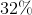off. If the dress originally cost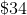, how much does it cost while it is on sale?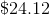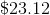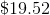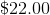Explanation:

Subtract the amount discounted from the original cost of the dress to find its sale price.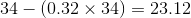The dress coston sale.

Example Question #10 : How To Find The Part From The Whole

Initially,number of students were going to chip in to buy their teacher a present that costdollars. Ifstudents decide not to pay, which of the following expressions represent how much each remaining student must now pay?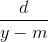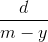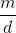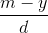Explanation:

To determine how much each person must pay, we will need to divide the total dollar amount by the number of people paying.

Before some students decided not to pay, the amount each person would have to pay is represented by the following expression: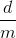Now, sincestudents dropped out, we need to subtract that from the number of people who will be paying.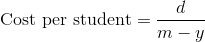Example Question #21 : Whole And Part

Pauline haspieces of candy. If she eatsof the candy then accidentally dropsof the remaining amount on the ground, how many pieces of candy does she have left?Explanation:

First, find out how many pieces of candy Pauline has after eatingof them.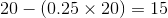Now, she losesof this remaining amount.  Since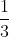of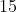is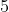, that means that her new total is 15 - 5 = 10 pieces of candy.

Pauline is left with 10 pieces of candy.

1 3 Next →